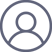余高奇2021-5-14 10:27

本文拟结合能量传递形式，讨论理想气体自由膨胀，并与经典热力学计算比较。

1. 问题

1摩尔理想气体氮气，在100kPa，298.15K下，自由膨胀至60kPa，计算该过程的WQ、ΔU及ΔS.

2. 解析

1845年，焦耳实验证实了理想气体自由膨胀为恒温过程；且该过程为理想气体pVT变化，有效功恒为0.

依题：上述过程参见图1.

Initial state1                                 Final state2

p1=100kPa，T1= 298.15K────→p2=60kPa, T2=298.15K

Fig.1 Process diagram

由理想气体状态方程可得：V=nRT/p

代入已知条件可得：V1=(1×8.314×298.15)/100=24.79dm3

V2=(1×8.314×298.15)/60=41.31dm3

2.1经典热力学

由于自由膨胀，外压pe=0, W=∫-pe▪dV=0

又由于该过程恒温，理想气体内能恒定，即：ΔU=0

依据热力学第一定律，ΔU=Q+W=0

所以：Q=0

另依热力学基本方程：dU=T▪dS-p▪dV  （1）

由式（1）可得：dS=（p/T）▪dV  =(nR/V)▪dV

积分可得：ΔS=nR▪ln(V2/V1)=8.314▪ln(41.31/24.79)=4.24J▪mol-1▪K-1

2.2 新观点

该过程有效功为0，依热力学基本方程可得：dU=T▪dS-p▪dV  （2）

理想气体恒温过程，内能保持恒定，即：dU=0

则：T▪dS-p▪dV =0

体势变WV=∫-p▪dV=∫(-nRT/V)▪dV=8.314×298.15▪ln(24.79/41.31)=-1.2658kJ▪mol-1

体积功WT=∫-pe▪dV=0

热量Q=∫T▪dS=-WV=1.2658kJ▪mol-1

恒温条件下，ΔS=Q/T=1.2658×103/298.15=4.24J▪mol-1▪K-1

2.3 结果对比

两种方法计算结果对比参见表1.

Tbl. 1 Comparison of calculation results of the two methods

─────────────────────────────────────

W/            Q /         ΔS /           ΔU/

(kJ/mol)    (kJ/mol)  [J/(mol▪K)]  (kJ/mol)

────────────────────────────────────────

Classical

thermodynamics            0              0            4.24              0

New point of view      -1.27         1.27          4.24            0

──────────────────────────────────

两种方法计算得到的内能变及熵变数值完全一致，不同之处在于对“功”的认知，经典热力学认为功即为体积功，体势变不存在；新观点认为：由于系统压强大于外压，体势变（-p▪dV ）占支配地位，体积功仅占体势变的一部分。

3. 结论

理想气体自由膨胀为恒温下的非绝热过程。

余高奇. 能量传递形式. 科学网博客，2021,4.